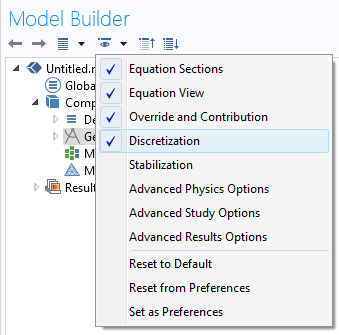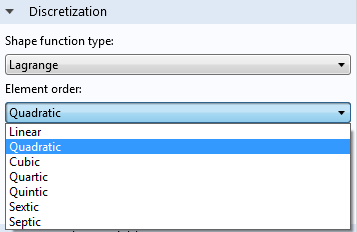# 弱形式的力量

2014年 4月 29日

### 如何从经典方程推导出弱方程

\nabla\cdot(-c\nabla u)=f

COMSOL 软件中的大多数方程都包含相似的项。对于传热应用，扩散系数 c，以及源项 f，分别指导热系数和热源。如果未知函数 u, 是原偏微分方程的解，也是以下积分方程的解：

\int_{\Omega}\nabla\cdot(-c\nabla u)v=\int_{\Omega}fv

\int_{\Omega}\nabla\cdot(-c\nabla u)v=\int_{\Omega}c\nabla u\cdot \nabla v+\int_{\partial \Omega}-c \nabla u\cdot n v

\int_{\Omega}c\nabla u\cdot \nabla v-\int_{\Omega}fv+\int_{\partial \Omega}-c \nabla u\cdot n v=0

### 试函数的选择选择离散化。在离散化设置中，默认使用拉格朗日单元。### COMSOL 语法中的弱方程

c*(ux*\mathrm{test}(ux)+uy*\mathrm{test}(uy)+uz*\mathrm{test}(uz))-f*\mathrm{test}(u)COMSOL Multiphysics 中提供了弱形式接口。### 弱方程的优势

• 是积分方程组的集合。网格决定了研究中求解的方程的数量。
• 允许为真实世界建模，因为它们都源自潜在的物理守恒定律。